# What Is The Law Of Sines? (5 Things You Need To Know)

The Law of Sines is useful in geometry and trigonometry when we solve triangles (to find their sides and angles).

So, what is the law of sines?  The Law of Sines relates the sides & angles of a triangle, using the sine function. If the triangle’s sides are a, b, & c, across from angles A, B, & C, then the Law of Sines tells us that a/sin(A) = b/sin(B) = c/sin(C). We can use this equation to solve for an unknown side or angle in a triangle.

Of course, there are some situations where we cannot use the Law of Sines.  In those cases, the Law of Cosines may be able to help us.

In this article, we’ll talk about the Law of Sines, what it is, and where it comes from.  We’ll also go through some examples so you know how to use the Law of Sines.

Let’s get started.

## What Is The Law Of Sines?

The Law of Sines relates the side lengths and angles of a triangle, using the sine trigonometric function.

Assume that a triangle has side lengths a, b, and c, with angles A, B, and C.  Side a is opposite angle A, side b is opposite angle B, and side c is opposite angle C, as shown below:To use the Law of Sines, we label the sides of a triangle as a, b, & c, with the opposite angles labeled A, B, & C.

The Law of Sines formula tells us that:

• a/sin(A) = b/sin(B) = c/sin(C)

We can also take the reciprocal to get the equations:

• sin(A)/a = sin(B)/b = sin(C)/c

### What Does The Law Of Sines Find?

The Law of Sines allows us to relate four numbers: any pair of sides and the corresponding pair of angles.

If we have any three of these numbers, we can use the Law of Sines to find the fourth one.  This process is known as triangulation.

## When To Use The Law Of Sines

The Law of Sines is used in two separate cases:

• 1. To find an unknown angle, given the side length opposite the unknown angle and another side-angle pair in the triangle.
• 2. To find an unknown side length, given the angle opposite the unknown side and another side-angle pair in the triangle.

Note that in either case, we must know one side-angle pair (a side length and the measure of the opposite angle), in addition to another side or angle, to use the Law of Sines.

### Can The Law Of Sines Be Used On Any Triangle?

The Law Of Sines can be used on any triangle.  As mentioned before, all we need is a side-angle pair and one other known quantity (a side length or an angle).The Law of Sines can be used on any triangle, including an acute triangle like this one (where each angle has a measure of less than 90 degrees).

The Law of Sines can help us to solve an acute, right, or obtuse triangle.  Note that for a right triangle, one of the angles is 90 degrees.

Let’s say that angle C is 90 degrees.  Then the Law of Sines formula becomes:

• a/sin(A) = b/sin(B) = c/sin(C)
• a/sin(A) = b/sin(B) = c/sin(90)
• a/sin(A) = b/sin(B) = c/1
• a/sin(A) = b/sin(B) = c

This tells us that for a right triangle (with C = 90), a = csin(A) and b = csin(B).

### How To Find An Angle Using The Law Of Sines

To find an angle using the Law of Sines, we must have:

• A side-angle pair (a side length and the measure of the opposite angle), plus another side length.

Let’s look at some examples to see how it works in practice.

#### Example 1: How To Find An Angles Using The Law Of Sines

Let’s say that we have the following triangle: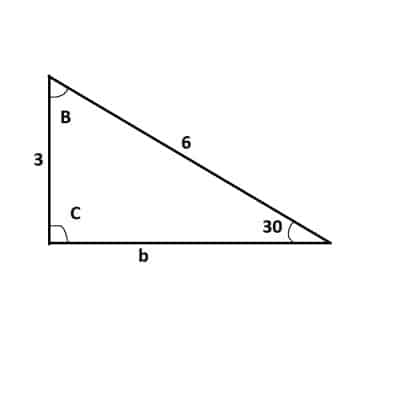We will use the Law of Sines with a = 3, A = 30, and c = 6 to solve this triangle.

By labeling the sides and angles, we can see that we have the side-angle pair a and A, along with side c.  Here, a = 3, A = 30, and c = 6.

First, we’ll find angle C.  Using the Law of Sines equation with these values gives us:

• a/sin(A) = c/sin(C)
• 3/sin(30) = 6/sin(C)
• 3/0.5 = 6/sin(C)
• 6 = 6/sin(C)
• 1 = 1/sin(C)
• sin(C) = 1
• C = 90

So, the measure of angle C is 90 degrees.

Since the angles in a triangle add up to 180, we can now find the measure of angle B:

• A + B + C = 180
• 30 + B + 90 = 180
• B + 120 = 180
• B = 60

So the measure of angle B is 60 degrees.

Since C = 90, this means that we have a right triangle.  We can use the Pythagorean Theorem to find side length b:

• a2 + b2 = c2
• a2 + b2 = c2
• 32 + b2 = 62
• 9 + b2 = 36
• b2 = 27
• b = √27
• b = 3√3

The full triangle is given below:

#### Example 2: How To Find An Angles Using The Law Of Sines

Let’s say that we have the following triangle: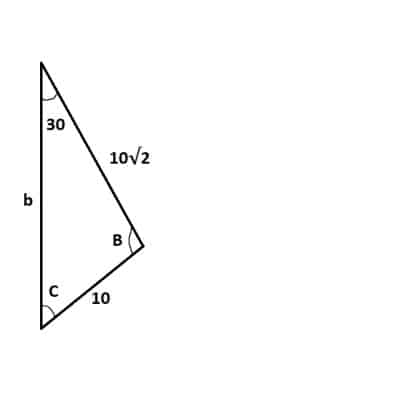We will use the Law of Sines with a = 10, A = 30, and c = 10√2 to solve this triangle.

By labeling the sides and angles, we can see that we have the side-angle pair a and A, along with side c.  Here, a = 10, A = 30, and c = 10√2.

First, we’ll find angle C.  Using the Law of Sines equation with these values gives us:

• a/sin(A) = c/sin(C)
• 10/sin(30) = 10√2/sin(C)
• 10/0.5 = 10√2/sin(C)
• 20 = 10√2/sin(C)
• 20/10√2 = 1/sin(C)
• 2/√2 = 1/sin(C)
• √2/2 = sin(C)
• C = 45

So, the measure of angle C is 45 degrees.  (Note: an angle of 135 degrees is also possible, since sin(135) is also √2/2.  This is an ambiguous case, which we will discuss more later).

Since the angles in a triangle add up to 180, we can now find the measure of angle B:

• A + B + C = 180
• 30 + B + 45 = 180
• B + 75 = 180
• B = 105

So the measure of angle B is 105 degrees.

Now we just need to find side length b.  We can use the Law of Sines again, this time including side b and angle B:

• a/sin(A) = b/sin(B)
• 10/sin(30) = b/sin(105)
• 10/0.5 = b/sin(105)
• 20 = b/sin(105)
• 20sin(105) = b

So the length of side b is 20sin(105), or approximately 20*(0.9659) = 19.3.

The full triangle is given below:

### How To Find A Missing Side Using The Law Of Sines

To find a missing side using the Law of Sines, we must have:

• A side-angle pair (a side length and the measure of the opposite angle), plus another angle measure.

Let’s look at some examples to see how it works in practice.

#### Example 1: How To Find A Missing Side Using The Law Of Sines

Let’s say that we have the following triangle: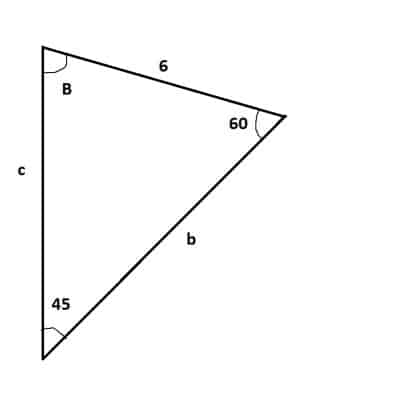We will use the Law of Sines with a = 6, A = 45, and C = 60 to solve this triangle.

By labeling the sides and angles, we can see that we have the side-angle pair a and A, along with angle C.  Here, a = 6, A = 45, and C = 60.

First, we’ll find side length c.  Using the Law of Sines equation with these values gives us:

• a/sin(A) = c/sin(C)
• 6/sin(45) = c/sin(60)
• 6*sin(60) = csin(45)  [cross multiply to eliminate fractions]
• 6*(√3/2)  = c*(√2/2)
• 6*(√3)  = c*(√2)  [multiply by 2 on both sides to eliminate fractions]
• 6*(√3)/ √2 = c
• 6√3√2/√2√2 = c
• 6√6/2 = c
• 3√6 = c

So, the side length c is 3√6.

Since the angles in a triangle add up to 180, we can now find the measure of angle B:

• A + B + C = 180
• 45 + B + 60 = 180
• B + 105 = 180
• B = 75

So the measure of angle B is 75 degrees.

Now we just need to find side length b.  We can use the Law of Sines again, this time including side b and angle B:

• a/sin(A) = b/sin(B)
• 6/sin(45) = b/sin(75)
• 6sin(75) = bsin(45)  [cross multiply to eliminate fractions]
• 6(0.9659) = b√2/2 [approximate value for sine of 75 degrees]
• 6(0.9659)*2/√2 = b
• 8.196 = b

So the length of side b is approximately 8.196.

The full triangle is given below:

## Why Does The Law Of Sines Work? (How To Prove The Law Of Sines)

The Law of Sines works because we can split any triangle into two right triangles and use the definition of the sine function to find the height.

Let’s say we have the following triangle: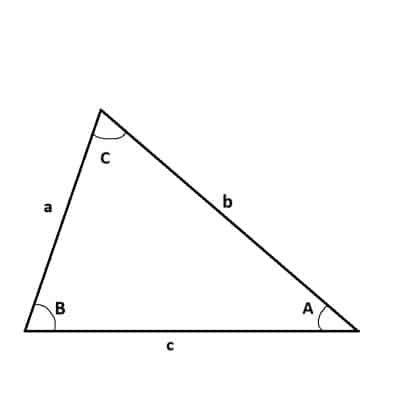To prove the Law of Sines, we will need a triangle with all sides and angles labeled.

First, we will draw a line segment from the vertex at angle C to side c, perpendicular to side c: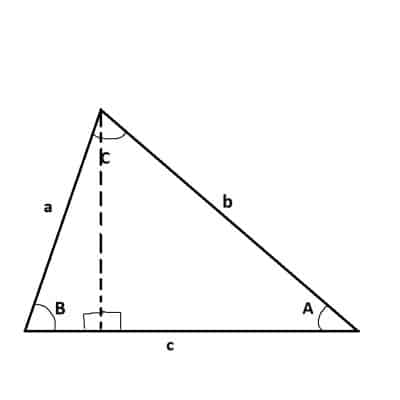Our first step is to draw a line segment from the vertex at angle C to side c (perpendicular to side C). This gives us two right triangles.

Next, we will label the perpendicular line segment H (the height of the triangle):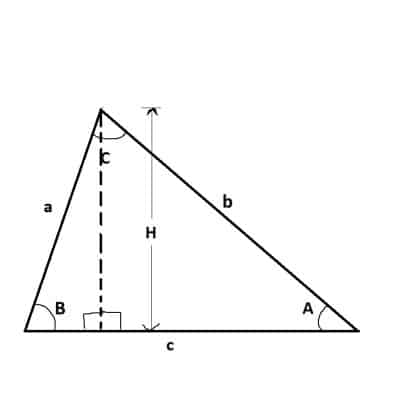Our next step is to label the height H of the triangle (the length of the perpendicular line segment we drew in the previous step).

Then, we will take the sine of angle A (opposite divided by hypotenuse) in the right-most right triangle to get:

• sin(A) = H/b

Similarly, we will take the sine of angle B (opposite divided by hypotenuse) in the left-most right triangle to get:

• sin(B) = H/a

Solving each of these equations for H gives us:

• H = bsin(A)
• H = asin(B)

Now, we set the two equations equal:

• H = H
• asin(B) = bsin(A)
• a/sin(A) = b/sin(B)

This is only part of the Law of Sines, but we can use a similar approach (draw a line segment from each of the other angles, perpendicular to the side opposite the angle) to get the other parts.

## What Is The Ambiguous Case For The Law Of Sines?

Sometimes, the values for a triangle’s sides and angles is not unique (that is, there is more than one possible set of sides and angles).  This is called the ambiguous case.

For example, in our earlier exercise, we found sin(C) = √2/2.  This has two possible solutions: C = 45 degrees and C = 135 degrees.

Without more information, we don’t know which angle to use.  Of course, we can split the problem into two cases and solve the triangle separately for C = 45 and also for C = 135.

## Conclusion

Now you know what the Law of Sines is and where it comes from.  You also know when to use the Law of Sines and how to apply the equation to find unknown sides or angles of a given triangle.